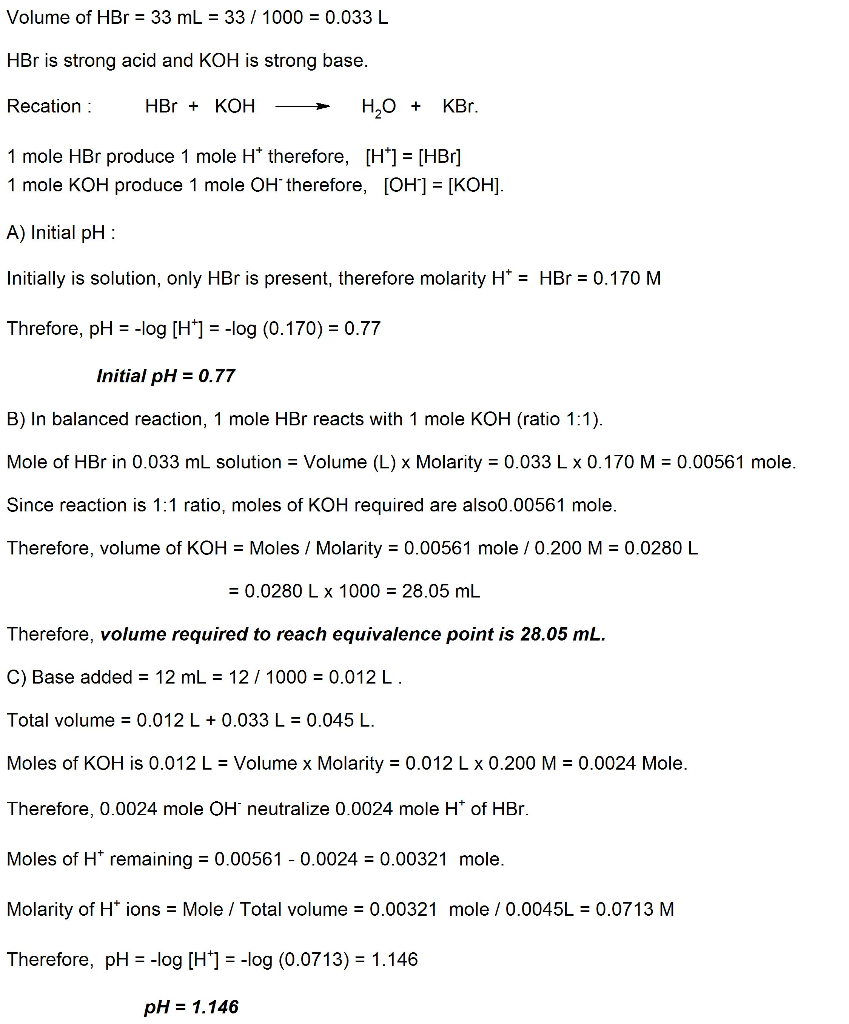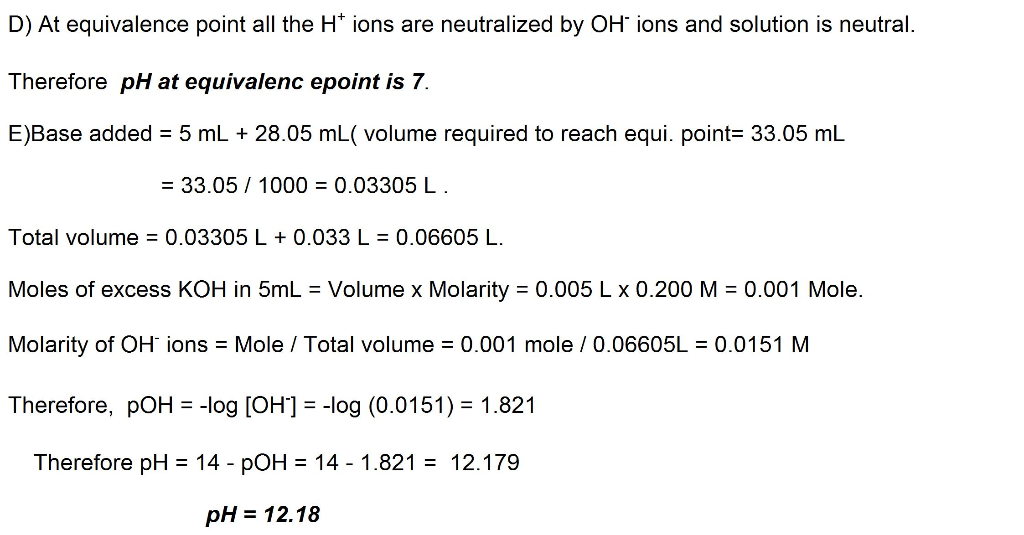Homework Help Question & Answers

# 7. Consider the titration of a 33.0 mL sample of 0.170 M  HBr with 0.200 M KOH. Determi...

7. Consider the titration of a 33.0 mL sample of 0.170 M  HBr with 0.200 M KOH. Determine each of the following:

A. the initial pH

B. the volume of added base required to reach the equivalence point (mL)

C. the pH at 12.0 mL of added base (express your answer using three decimal places.)

D. the pH at the equivalence point (express your answer as a whole number.)

E. the pH after adding 5.0 mL of base beyond the equivalence point (express your answer using two decimal places)

#### Homework Answers

ReportAnswer #1Add Homework Help Answer
##### Add Answer of: 7. Consider the titration of a 33.0 mL sample of 0.170 M  HBr with 0.200 M KOH. Determi...
Your Answer: Your Name: What's your source?
Not the answer you're looking for? Ask your own homework help question. Our experts will answer your question WITHIN MINUTES for Free.
More Homework Help Questions Additional questions in this topic.

• #### Nonparametrics Testing baseline 180 136 210 170 140 160 150 110 120 125 230 124 110 164 170 106 1...

Need Online Homework Help?

Get FREE EXPERT Answers
WITHIN MINUTES
Related Questions